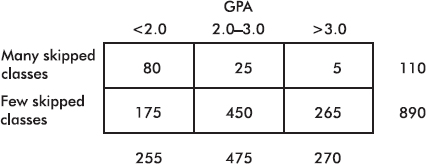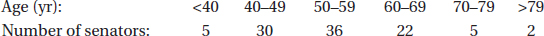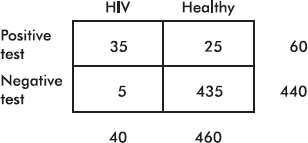# AP Statistics Multiple-Choice Practice Questions: Probability as Relative Frequency 3

### Test Information12 questions28 minutes

1. Question below refers to the following study: One thousand students at a city high school were classified both according to GPA and whether or not they consistently skipped classes.What is the probability that a student has a GPA under 2.0 and has skipped many classes?

2. Question below refers to the following study: One thousand students at a city high school were classified both according to GPA and whether or not they consistently skipped classes.What is the probability that a student has a GPA under 2.0 or has skipped many classes?

3. Question below refers to the following study: One thousand students at a city high school were classified both according to GPA and whether or not they consistently skipped classes.What is the probability that a student has a GPA under 2.0 given that he has skipped many classes?

4. Question below refers to the following study: One thousand students at a city high school were classified both according to GPA and whether or not they consistently skipped classes.Are "GPA between 2.0 and 3.0" and "skipped few classes" independent?

5. Mathematically speaking, casinos and life insurance companies make a profit because of

6. Consider the following table of ages of U.S. senators:What is the probability that a senator is under 70 years old given that he or she is at least 50 years old?

7. Question below refers to the following study: Five hundred people used a home test for HIV, and then all underwent more conclusive hospital testing. The accuracy of the home test was evidenced in the following table.What is the predictive value of the test? That is, what is the probability that a person has HIV and tests positive?

8. Question below refers to the following study: Five hundred people used a home test for HIV, and then all underwent more conclusive hospital testing. The accuracy of the home test was evidenced in the following table.What is the false-positive rate? That is, what is the probability of testing positive given that the person does not have HIV?

9. Question below refers to the following study: Five hundred people used a home test for HIV, and then all underwent more conclusive hospital testing. The accuracy of the home test was evidenced in the following table.What is the sensitivity of the test? That is, what is the probability of testing positive given that the person has HIV?

10. Question below refers to the following study: Five hundred people used a home test for HIV, and then all underwent more conclusive hospital testing. The accuracy of the home test was evidenced in the following table.What is the specificity of the test? That is, what is the probability of testing negative given that the person does not have HIV?

11. Suppose that 2% of a clinic's patients are known to have cancer. A blood test is developed that is positive in 98% of patients with cancer but is also positive in 3% of patients who do not have cancer. If a person who is chosen at random from the clinic's patients is given the test and it comes out positive, what is the probability that the person actually has cancer?

12. Suppose the probability that you will receive an A in AP Statistics is 0.35, the probability that you will receive A's in both AP Statistics and AP Biology is 0.19, and the probability that you will receive an A in AP Statistics but not in AP Biology is 0.17. Which of the following is a proper conclusion?